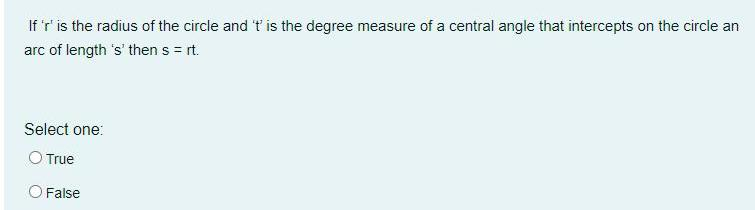Question:

# If 'r' is the radius of the circle and 't' is the degree

Last updated: 7/4/2022If 'r' is the radius of the circle and 't' is the degree measure of a central angle that intercepts on the circle an arc of length 's' then s = rt. Select one: O True O False